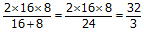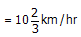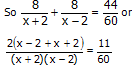CUET  >  Boats And Streams

# Boats And Streams - General Test Preparation for CUET

Chapter - 17

BOATS AND STREAMS

POINTS TO KNOW AND REMEMBER

i) When speed of boat is given, it means the speed of the boat in still water.

ii) Upstream means that the boat or a person in moving against the flow of water and downstream means in the direction of the flow of water.

iii) if x and y are the speeds of the boat and speed of the stream / river respectively then the relative or effective speed of boat with respect to the stream if it moves upstream is x – y but down stream it will be x + y

SOLVED EXAMPLES

Example 1. In one hour, a boat goes 11 km along the stream and 5 km against the stream. The speed of the boat in still water is

• Let x be the speed of boat in still water and y be the speed of the stream

then x + y = 11

x – y = 5 or 2x = 16or x = 8km/hr.

shortcut = Add the two speed, and divide by 2

i.e. speed of boat in still water = (11 +5) / 2 = 8 km/per hr.

Example 2: A man can row upstream at 10 km/hr and down stream 16 km/hr the speed of the stream is?

• Let x be the speed of boat and y be the speed of the stream.

Then x + y = 16

X – y = 10 or 2x = 26 x = 13

2y = 6

y = 3

Speed of stream 3 km/hr

Shortcut: the speed of stream is (x – y) / 2

or (16 – 10) / 2 = 3 km/hr

Example 3: A man rows upstream 32 km and down stream 17 km. If to row the same distance upstream and down stream he takes 5 hours (in each direction) the velocity of the current is

•  if x is the speed of the man

And y is the speed of the current

The (x – y) / 2 is the distance travelled by the stream in 5 hours.

Or (32 – 17) / 2 = 15 / 2 = 7.5 km

So the speed of the current is 7.5/5 = 1 ½ km/hr

Example 4: There is a road beside a river. Two friends start from a place A and reach a point B and come back again to A. One of them moves at a speed of 12 km/hr on a bicycle while the other sails on a boat at a speed of 12 km/hr. If the river flows at a rate of 4 km/hr which of the two friends will reach to place A first.

Since the cyclist moves both ways at the speed of 12 km/hr. so his average speed is 12 km/hr.

The boat sailor moves downstream at a speed of 12 + 4 = 16 km/hr

And moves upstream at a speed of 12 – 4 = 8 km/hr

His average speed can be found by Harmonic mean

Average speed =Since the average speed of the cyclist is more so he will reach back at A first.

5. Speed of a stream is 2 km/hr. A motor boat goes upstream upto a distance of 8 km upstream and reaches back at the starting point in 44 minutes. Find the speed of the motor boat in still water.

• let the speed of the motor boat in still water be x

So speed upstream = x – 2

Speed down stream = x + 2Or 240 x = 11 (x + 2) (x – 2)

Or 240 x = 11x2 – 44

Or 11x2 – 240x – 44 = 0

Or (11x + 2) (x – 22) = 0

Or x = 22

So speed of the motor boat in still water = 22 km/hr

6. The speed of a boat in still water is 10 km/hr. if it can travel 26 km down stream and 14 km upstream in the same time, what is the speed of the stream.

• Let the speed of stream be x km/hr. Speed down stream is (10 + x) km/hr

Speed up stream is (10 – x) km/hr

So 26/(10+x) =14/(10-x) or 260-26 x = 140+14x

Or 40 x = 120 x = 3

So speed of stream is 3 km/hr

The document Boats And Streams | General Test Preparation for CUET is a part of the CUET Course General Test Preparation for CUET.
All you need of CUET at this link: CUET

## General Test Preparation for CUET

177 videos|326 docs|399 tests

## FAQs on Boats And Streams - General Test Preparation for CUET

 1. What are boats and streams?Ans. Boats and streams refer to a concept in mathematics that deals with the relative speed of a boat or a person in still water and the speed of the stream. It involves calculating the speed of the boat or person in still water and the speed of the stream to determine their effective speed.
 2. How can I calculate the speed of a boat or person in still water?Ans. To calculate the speed of a boat or person in still water, you need to know the distance covered and the time taken. Divide the distance by the time to get the speed. However, if the speed of the stream is known, you need to subtract it from the calculated speed to get the speed in still water.
 3. How do I calculate the speed of the stream?Ans. To calculate the speed of the stream, you need to know the distance covered and the time taken when moving downstream and upstream. Subtract the speed of the boat or person in still water from the speed calculated when moving downstream to get the speed of the stream. Similarly, add the speed of the boat or person in still water to the speed calculated when moving upstream to get the speed of the stream.
 4. Can you explain how to solve boat and stream problems involving multiple speeds and distances?Ans. Yes, in boat and stream problems involving multiple speeds and distances, you can break down the given scenario into smaller parts. Calculate the effective speed of the boat or person in still water for each part and then add or subtract the speed of the stream accordingly. Finally, you can combine the individual speeds to get the overall speed or time taken.
 5. Are boats and streams used in real-life scenarios?Ans. While boats and streams are primarily used in mathematical problems, the concept can be applied to real-life scenarios as well. For example, it can be used to calculate the effective speed of a boat or person in a river with a current. It can also be useful for understanding the impact of the stream's speed on navigation or swimming against the current.

## General Test Preparation for CUET

177 videos|326 docs|399 testsExplore Courses for CUET examSignup to see your scores go up within 7 days! Learn & Practice with 1000+ FREE Notes, Videos & Tests.
10M+ students study on EduRev
Track your progress, build streaks, highlight & save important lessons and more!
Related Searches

,

,

,

,

,

,

,

,

,

,

,

,

,

,

,

,

,

,

,

,

,

;